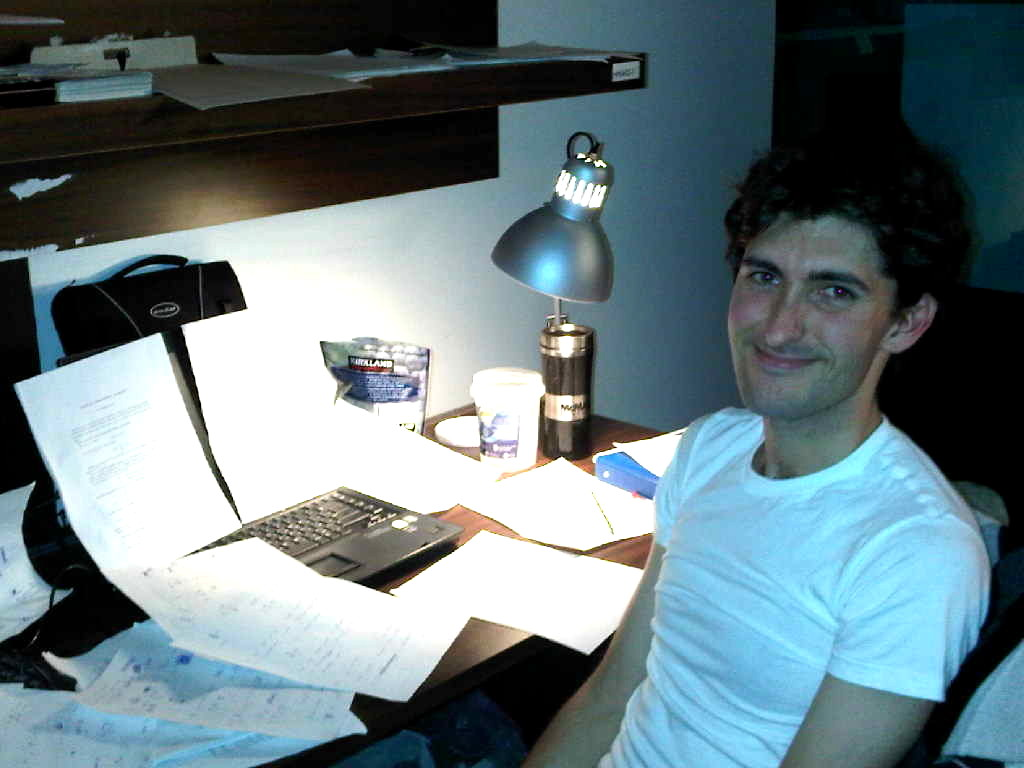Dr.  Dmitry Ponomarev
Email: dmvpon@gmail.com / dmitry.ponomarev@asc.tuwien.ac.at,
Office DA 06 L14, Institute of Analysis & Scientific Computing,
Vienna University of Technology (TU Vienna),
Wiedner Hauptstrasse 8, 1040 Vienna, Austria.### Papers in preparation:

• "Revisiting the limiting amplitude principle for the wave equation with variable coefficients" (with A. Arnold, S. Geevers, I. Perugia).

• "On a transformation of Schrödinger equation to a simple first order equation with complex conjugation: theoretical and practical applications".

• "On some integral equations pertinent to the punch problem with wear" (with I. Argatov).

• "Recovery of magnetization features from partial field measurements: Fourier and Kelvin transforms approaches" (with J. Leblond, L. Baratchart, E. Lima).

• "On asymptotic extension of convolution theorem for Fourier cosine transform for certain integral equations on half-line".

• "New method for construction of asymptotic solution for Prandtl lifting line equation".